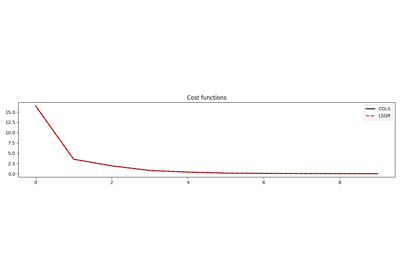# pylops.optimization.solver.cgls¶

pylops.optimization.solver.cgls(Op, y, x0, niter=10, damp=0.0, tol=0.0001, show=False, callback=None)[source]

Conjugate gradient least squares

Solve an overdetermined system of equations given an operator Op and data y using conjugate gradient iterations.

Parameters: Op : pylops.LinearOperator Operator to invert of size $$[N \times M]$$ y : np.ndarray Data of size $$[N \times 1]$$ x0 : np.ndarray, optional Initial guess niter : int, optional Number of iterations damp : float, optional Damping coefficient tol : float, optional Tolerance on residual norm show : bool, optional Display iterations log callback : callable, optional Function with signature (callback(x)) to call after each iteration where x is the current model vector x : np.ndarray Estimated model of size $$[M \times 1]$$ istop : int Gives the reason for termination 1 means $$\mathbf{x}$$ is an approximate solution to $$\mathbf{d} = \mathbf{Op}\,\mathbf{x}$$ 2 means $$\mathbf{x}$$ approximately solves the least-squares problem iit : int Iteration number upon termination r1norm : float $$||\mathbf{r}||_2$$, where $$\mathbf{r} = \mathbf{d} - \mathbf{Op}\,\mathbf{x}$$ r2norm : float $$\sqrt{\mathbf{r}^T\mathbf{r} + \epsilon^2 \mathbf{x}^T\mathbf{x}}$$. Equal to r1norm if $$\epsilon=0$$ cost : numpy.ndarray, optional History of r1norm through iterations

Notes

Minimize the following functional using conjugate gradient iterations:

$J = || \mathbf{y} - \mathbf{Opx} ||_2^2 + \epsilon^2 || \mathbf{x} ||_2^2$

where $$\epsilon$$ is the damping coefficient.

## Examples using pylops.optimization.solver.cgls¶CGLS and LSQR Solvers